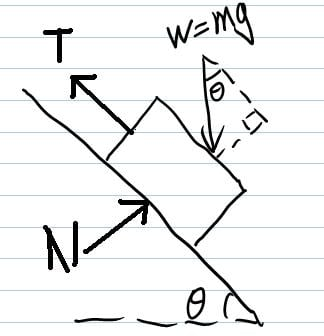# Weight component

spdowind

## Homework Statement

Well I am trying to get help on weight compent. It is a box sliding on an inclined surface with angle 30 degrees.

w= mg

## The Attempt at a Solution

I am trying to find the components for weight force acting on the box. Book says w=mgcos(30) is when its perpendicular to surface, and w= mgsin(30) when its parallel to surface. Can someone explain how to do this?
Thanks

## Answers and Replies

Homework Helper
Gold Member
I am trying to find the components for weight force acting on the box. Book says w=mgcos(30) is when its perpendicular to surface, and w= mgsin(30) when its parallel to surface. Can someone explain how to do this?
Thanks
spdowind,welcome to PF! Are you familiar with the method for calculating vector components by drawing a right triangle? Draw a sketch. The weight force acts straight down vertically....that's the hypotenuse of the right triangle. The components are the magnitudes of the legs of that right triangle, one leg parallel to the plane surface, and the other perperpendicular to it. You'll have to do a little geometry and some basic trig to calculate these components. Please show some attempt at this for further assistance.

spdowindSee i am trying to find the horizontal forces for the block sliding down.
I did:
Fx= m* ax
mg*sin(angle)- Tension force - Friction Force= mass * Acceleration.

I just dont understand why I use mg* sin ( angle) instead of mg*cos(angle) when I do the weight x-component minus tension force and friction force.

spdowind
well from my understand again,my graph is a lil off the angle is wrong.
What I do understand is:

The weight is split into 2 components like my graph, but one is horizontal to the sliding surface , one is perpendicular to it, the one horizontal is the X compoent, which means parallel to surface means mgsin(angle)? Am I on the right track? Because I set up my x-y coordinates horizontal to sliding surface and perpendicular to surface.

Thanks